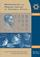Középiskolai Matematikai és Fizikai Lapok
Informatika rovattal
 Már regisztráltál? Új vendég vagy?# Angol nyelvű szám, 2002. december

Előző oldalTartalomjegyzékKövetkező oldalMEGRENDELŐLAP

## Solution of the problems for information technology November 2001

I. 9. Binomial coefficients can be arranged in the usual Pascal's triangle as in the figure. Except for the outermost entries in each row, every number is the sum of its upper and upper-left neighbours.

 1 N=6 1 1 1 2 1 1 3 3 1 1 4 6 4 1 1 5 10 10 5 1 1 6 15 20 15 6 1

Prepare your Excel sheet capable of displaying the first N+1 rows of Pascal's triangle in this arrangement. Let the value of N (1 $\displaystyle \le$N $\displaystyle \le$20) be entered in the 7th cell of the first row. The sheet should contain exactly N+1 rows in every case.

(10 points)

Solution. The solution is simple taking into account the well-known properties of the Pascal's triangle: any element is the sum of its upper neighbours. But our table is on a tilt now, so its upper and upper-left neighbours are to be summed.

In column

A:=IF(ROW()<=$H$1+1;1;""),

there is everywhere either 1's or nothing, corresponding to the N+1 rows to be displayed, that is depending on the number of the row.

The other cells will contain

=IF(ROW()<=$H$1+1;C5+D5;""),

since only those rows are to be printed here in which the number of the row is at most N+1 (the value of N is at . In our example, according to the formula in cell D6, the values of cell D5 and C5 should be added.

Filling of the table is also simple, because Excel automatically increases the row and column identifiers of the cells upon filling. One has to take care not to put anything into cells from the sixth one in the second row, otherwise the whole triangle will be incorrect: we can not carry out addition using only one character.